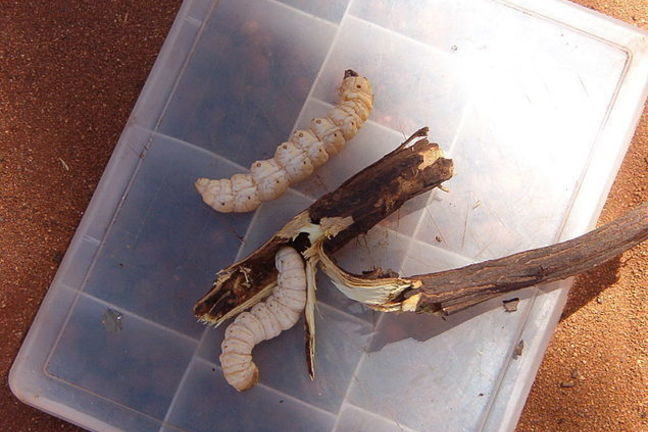## Want to keep learning?

This content is taken from the UNSW Sydney's online course, Maths for Humans: Linear, Quadratic & Inverse Relations. Join the course to learn more.
1.5

## UNSW Sydney# Australian bush tucker

Adventurer Bear Grylls has claimed that, “Pound for pound, insects contain more protein than beef”. Let’s investigate this claim!

In this lesson, we learn

• how to convert between different units: pound to grams, witchetty grub to protein and protein to witchetty grub
• how to use proportionality to find out how much witchetty grub do you need to satisfy your daily protein needs?
• that insects are an undervalued food group.

## Where is the protein?

There are few cows in the deserts of outback Australia. In fact, prior to European settlement in 1788 there were exactly zero cows. So what did people eat? Well, they actually had a pretty healthy diet which included… insects.

“Insects represent a genuine food category – their consumption is by no means a mere emergency measure”

Meyer-Rochow 1976.

This quote is especially true for the witchetty grub because of its high nutritional value and delicious flavor. Bear Grylls makes the case that insects contain more protein than beef. Let’s see if this claim is supported by evidence!

## Convert witchetty grubs to protein

Dried witchetty grub larva is about $$\normalsize{38\%}$$ protein. The relation between witchetty grubs and protein is given by the equation:

$\Large{ \text{1 gram of witchetty grub} = \frac{38}{100} \text{ grams of protein.} \label{w2p} \tag{1}}$

Or, in terms of pounds

$\Large{\text{1 pound of witchetty grub} = \frac{38}{100} \text{ pounds of protein.}}$

Beef, in comparison, is only $$\normalsize{14\%}$$ protein.

$\Large{\text{1 gram of beef} = \frac{14}{100} \text{ grams of protein.} \label{b2p} \tag{2}}$

Again, in terms of pounds

$\Large{ \text{1 pound of beef} = \frac{14}{100} \text{ pounds of protein.}}$

Now let’s look at Bear Gryll’s claim. One pound of witchetty grub contains $$\normalsize{0.38}$$ pounds of protein, compared to one pound of beef, which contains $$\normalsize{0.14}$$ pounds of protein. Since

$\Large{ \begin{equation*} 0.38 > 0.14, \end{equation*} }$

We conclude that pound for pound, witchetty grub contains more protein than beef! So this claim holds up under closer mathematical scrutiny. Thanks for the dietary advice, Mr Grylls.

## Convert protein to witchetty grubs

How many witchetty grubs do you need to eat in order to obtain one gram of protein? We can obtain this information by rearranging the equation $$\normalsize{(\ref{w2p})}$$ into:

$\Large{\text{1 gram of protein} = \frac{100}{38} \text{ grams of witchetty grub.} \label{p2g}\tag{3} }$

So to obtain one gram of protein, you need to eat $$\normalsize{\frac{100}{38} \approx 2.62}$$ grams of witchetty grubs.

Q1 (E): How many grams of beef do you need to eat in order to obtain one gram of protein?

## How much witchetty grub does your little brother or sister need?

Your daily protein requirements vary. For example, school aged children require roughly $$\normalsize{26}$$ grams of protein every day. So how much witchetty grub do school aged children require? We use equation $$\normalsize{(\ref{p2g})}$$ as follows:

\Large{ \begin{align} 26 \text{ grams of protein} & = 26 \times \frac{100}{38} \text{ grams of witchetty grub} \\ & \approx 68.42 \text{ grams of witchetty grub.} \end{align} }

## How much witchetty grub do you need?

Q2 (C): Before you introduce witchetty grub to your diet, you should know that protein requirements increase with age. Use an internet search to find out your daily recommended protein intake, and then let us know how much witchetty grub you need per day in the comments. It might help to know that one whole dried larval witchetty grub weighs about $$\normalsize{0.78}$$ grams.

## Discussion

Aboriginal Australians were certainly not the only groups to appreciate the nutritional value of insects as a food source. Can you find some other interesting examples, and perhaps share some protein/gram statistics?

A1. Rearrange equation $$\normalsize{(\ref{b2p})}$$ into

$\Large{ \text{1 gram of protein} = \frac{100}{14} \text{ grams of Beef.}}$

So the answer is about $$\normalsize{7.14}$$ grams of Beef.

A2. If my recommended daily protein intake is $$\normalsize{50}$$ grams, then I need

$\Large{\begin{array}{rll} 50 \text{ grams of protein} = & 50 \times \frac{100}{38} & \text{ grams of witchetty grub} \\ \approx & 131.58 & \text{ grams of witchetty grub} \\ = & \frac{131.58}{0.78} & (0.78 \text{ grams of witchetty grub}) \\ = & \frac{131.58}{0.78} & \text{ whole dried witchetty grub} \\ \approx & 168.69 & \text{ whole dried witchetty grub.} \end{array} }$

Meyer-Rochow, V.B. 1976. The use of insects as human food. Food and Nutr.; Notes and Reviews 33(4): 151-152. (Introduction)